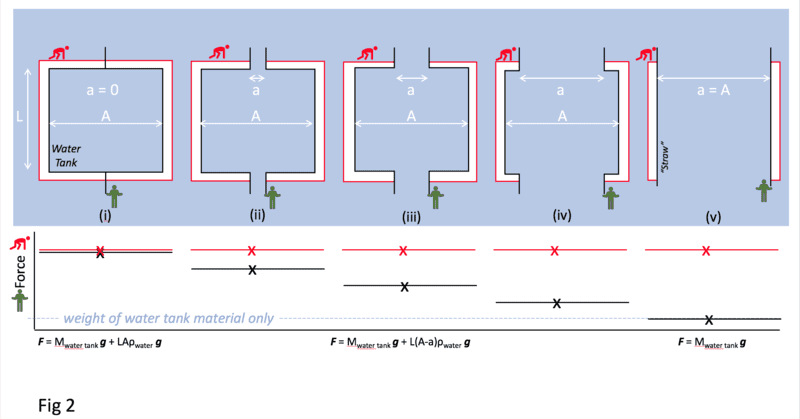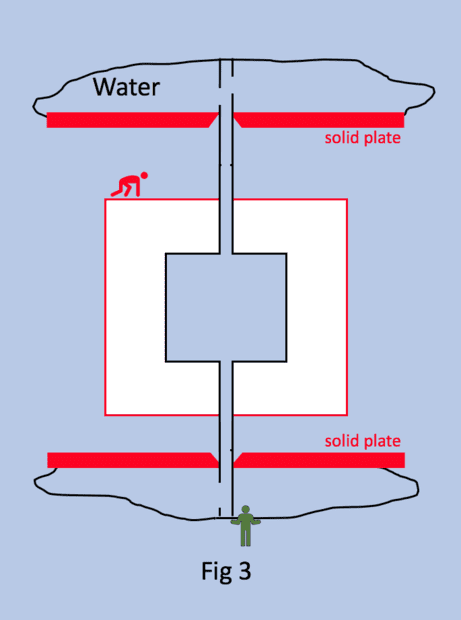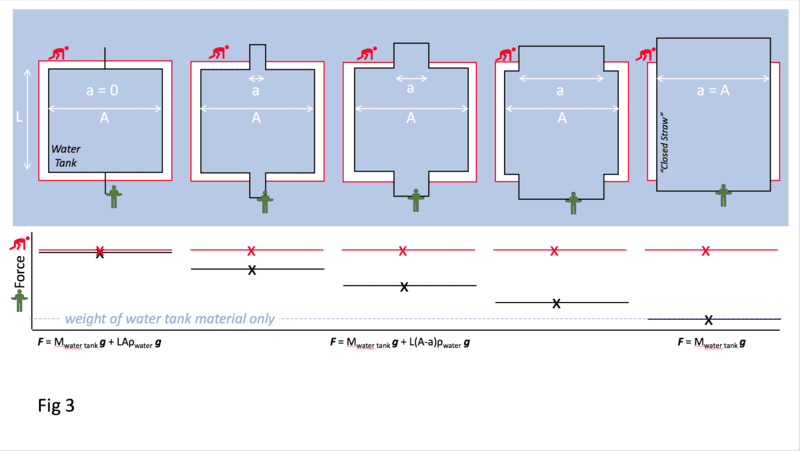# Buoyancy and External Forces

Richard Spiteri
What I'm saying, to be more precise, is that the green guy exerts an upward force given by:
$$F=M_{water\ tank}g+L(W-w)\rho_{water}g$$where w is the width of the tube opening (where the water is present in each tube). In this way, only when W = w (the tank width is equal to the tube width), ##F=M_{water\ tank}g##, but otherwise, no.

@Chestermiller , This is your equation as relates to my original Fig 2.

F = Mwater tankg + L(A-ag

(Note, @sophiecentaur , that I changed W to A and w to a so there is no confusion with upper case and lower case fonts and to reflect 'areas' rather than '2D widths'.)

I "plotted" (more like sketched) the net force that the Green man exerts to show that it changes from lifting the total weight of the tank material plus water (water tank on the left) to the weight of the material with no water (straw on the right).

I now understand this problem and I agree (thank you @Chestermiller ).

Please see my next post (#37) as relates to my original Fig 3 with water bags isolating the hollow tubes.#### Attachments

Last edited:
Richard SpiteriThe figure above, is my original Fig 3 reposted for clarity

In Fig 3, I isolated the hollow tubes from the outside water using the water bags. My question was "what force is the Green man exerting in each of the cases below" as the width a of the hollow tube increases from 0 to A?The figure above with the accompanying 'sketched' plot shows my thoughts on the net force F that the Green guy exerts versus the width a of the closed hollow tube. I believe this is correct (i.e., the same results as Fig 2). Is this right?

#### Attachments

Last edited:
Richard Spiteri
Also, what is the upthrust U (that the Red man must resist) on the Air Tank in #37?

Personally, I think it is a constant equal to the volume of water that the Air Tank displaces

U = LAir Tank . AAir Tankwaterg

if you assume that the Air tank dimensions are LAir Tank by AAir Tank.

Or am I counting the volume contributions of air tank and water tank twice (ref @kuruman where we must either include the volume and weight of the water everywhere or we must exclude the volume and weight of the water everywhere)?

Also, since I assumed that the relation between the Air Tank and the Water Tank is frictionless and water tight, then the net upwards force on the Air tank and Water Tank together must be equal to the difference in the two forces on my plot (actually the sum but taking into account that they are in opposite directions).

Last edited:
Mentor
I get that the downward force that the red guy must exert is $$F=L(A_{air\ tank}-a)\rho_{water}g-M_{air\ tank}g$$Does this make sense?